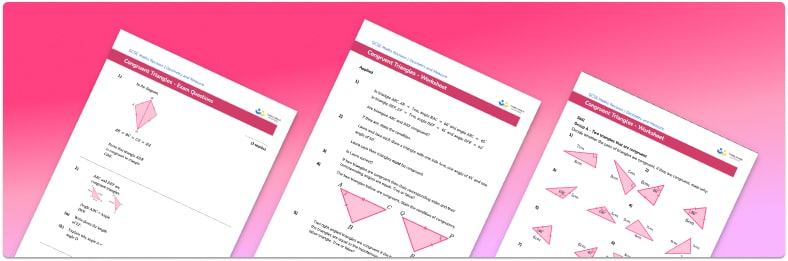# Congruent Triangles Worksheet• Section 1 of the congruent triangles worksheet contains 20+ skills-based congruent triangles questions, in 3 groups to support differentiation
• Section 2 contains 3 applied congruent triangles questions with a mix of worded problems and deeper problem solving questions
• Section 3 contains 3 foundation and higher level GCSE exam style congruent triangles questions
• Answers and a mark scheme for all congruent triangles questions are provided
• Questions follow variation theory with plenty of opportunities for students to work independently at their own level
• All questions created by fully qualified expert secondary maths teachers
• Suitable for GCSE maths revision for AQA, OCR and Edexcel exam boards

• This field is for validation purposes and should be left unchanged.

You can unsubscribe at any time (each email we send will contain an easy way to unsubscribe). To find out more about how we use your data, see our privacy policy.

### Congruent triangles at a glance

Congruent triangles are triangles which are exactly the same. If two triangles are congruent, all of their sides and angles are the same as each other. There are four ways to prove triangle congruence. These are:

ASA – if you can show that two sides and the angle between them are the same in both triangles, they must be congruent
SAS – if you can show that two angles and the side between them are the same in both triangles they must be congruent
SSS – if all three sides of the triangles are the same, they are congruent
RHS – if the triangles are right angle triangles with equal hypotenuses and one other equal side, they must be congruent

Take a parallelogram, ABCD where AC and BD are opposing vertices and the diagonal AC is connected, triangulating the parallelogram.

Triangle ABC and triangle ADC are a pair of congruent triangles. Since opposite sides in a parallelogram are equal, BC and AD are corresponding sides in the two triangles. The triangles also share the side AC, the diagonal of the parallelogram. Finally, angles BCA and CAD are alternate angles meaning they are equal. The triangles are congruent by SAS.

When proving congruence, we often need to use a variety of angle facts to identify whether angles are equal. These angle facts might  include angles in a triangle, angles in parallel lines and circle theorems

Looking forward, students can then progress to additional geometry worksheets, for example a simplifying expressions worksheet or simultaneous equations worksheet.For more teaching and learning support on Geometry our GCSE maths lessons provide step by step support for all GCSE maths concepts.

## Do you have KS4 students who need more focused attention to succeed at GCSE?There will be students in your class who require individual attention to help them succeed in their maths GCSEs. In a class of 30, it’s not always easy to provide.

Help your students feel confident with exam-style questions and the strategies they’ll need to answer them correctly with our dedicated GCSE maths revision programme.

Lessons are selected to provide support where each student needs it most, and specially-trained GCSE maths tutors adapt the pitch and pace of each lesson. This ensures a personalised revision programme that raises grades and boosts confidence.

Find out more